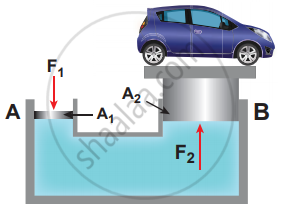Tamil Nadu Board of Secondary EducationHSC Science Class 11th

# State and prove Pascal’s law in fluids. - Physics

State and prove Pascal’s law in fluids.

#### Solution

Statement of Pascal’s law: If the pressure in a liquid is changed at a particular point, the change is transmitted to the entire liquid without being diminished in magnitude.Hydraulic lift

Application of Pascal’s law:

Hydraulic lift: A practical application of Pascal’s law is the hydraulic lift which is used to lift a heavy load with a small force. It is a force Hydraulic lift multiplier. In consists of two cylinders, A and B connected to each other by a horizontal pipe, filled with a liquid. They are fitted with frictionless pistons of cross-sectional areas A1 and A2 (A2 > A1). Suppose a downward force F is applied on the smaller piston, the pressure of the liquid under this piston increases to "P"("where", "P" = "F"_1/"A"_1). But according to Pascal’s law, this increased pressure P is transmitted undiminished in all directions. So pressure is exerted on piston B. Upward force on piston B is

"F"_2 = "P" xx "A"_2 = "F"_1/"A"_1 xx "A"_2 ⇒ "F"_2 = "A"_2/"A"_1 xx "F"_1

Therefore by changing the force on the smaller piston A, the force on the piston B has been increased by the factor "A"_2/"A"_1 and this factor is called the mechanical advantage of the lift.

Concept: Fluids
Is there an error in this question or solution?

#### APPEARS IN

Tamil Nadu Board Samacheer Kalvi Class 11th Physics Volume 1 and 2 Answers Guide
Chapter 7 Properties of Matter
Evaluation | Q III. 5. | Page 92
Share